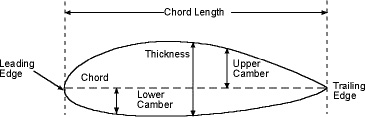A# chord (of an airfoil)In aerodynamics, a chord is a line that extends from the leading edge to the trailing edge of an airfoil. The chord is a basic reference axis for the geometric or aerodynamic properties of an airfoil. It is normal to the span and lies in the plane of the airfoil.

There are two of these reference chords. The one used for general and structural reference is the geometric chord. The other is the aerodynamic chord, being an imaginary line through the airfoil parallel to the free air stream at zero lift and passing through the trailing edge. The length of this chord is of no importance. It is useful mainly in aerodynamic studies because the lift varies directly with the angle of attack of the aerodynamic chord.

If the airfoil has a flat lower surface, an element of this surface is taken as the geometric chord. The chord length is the overall projection of the profile on this chord. In double-cambered airfoils the geometric chord is taken as the longest straight line possible between leading and trailing edges, or as a straight line joining the ends of the profile median line. The angle of attack to the geometric chord at zero lift is the angle between these chords. This may be discovered by wind tunnel tests, although empirical constructions have been devised which locate the aerodynamic chord surprisingly well.

If the wing is tapered there is a tip chord and a root chord. The location of the intermediate chord of which the aerodynamic forces could be assumed to act is called the mean aerodynamic chord and is important in studies of airplane balance and stability. When the coefficient of lift may be assumed to be constant over the semispan, the mean aerodynamic chord coincides with the mean geometric chord (i.e., the centroid of the semiwing plan form.) This simplification is in error if the wing has a twist, of if it is rectangular, in which case the uneven downwash causes decreased lift coefficient near the tips.